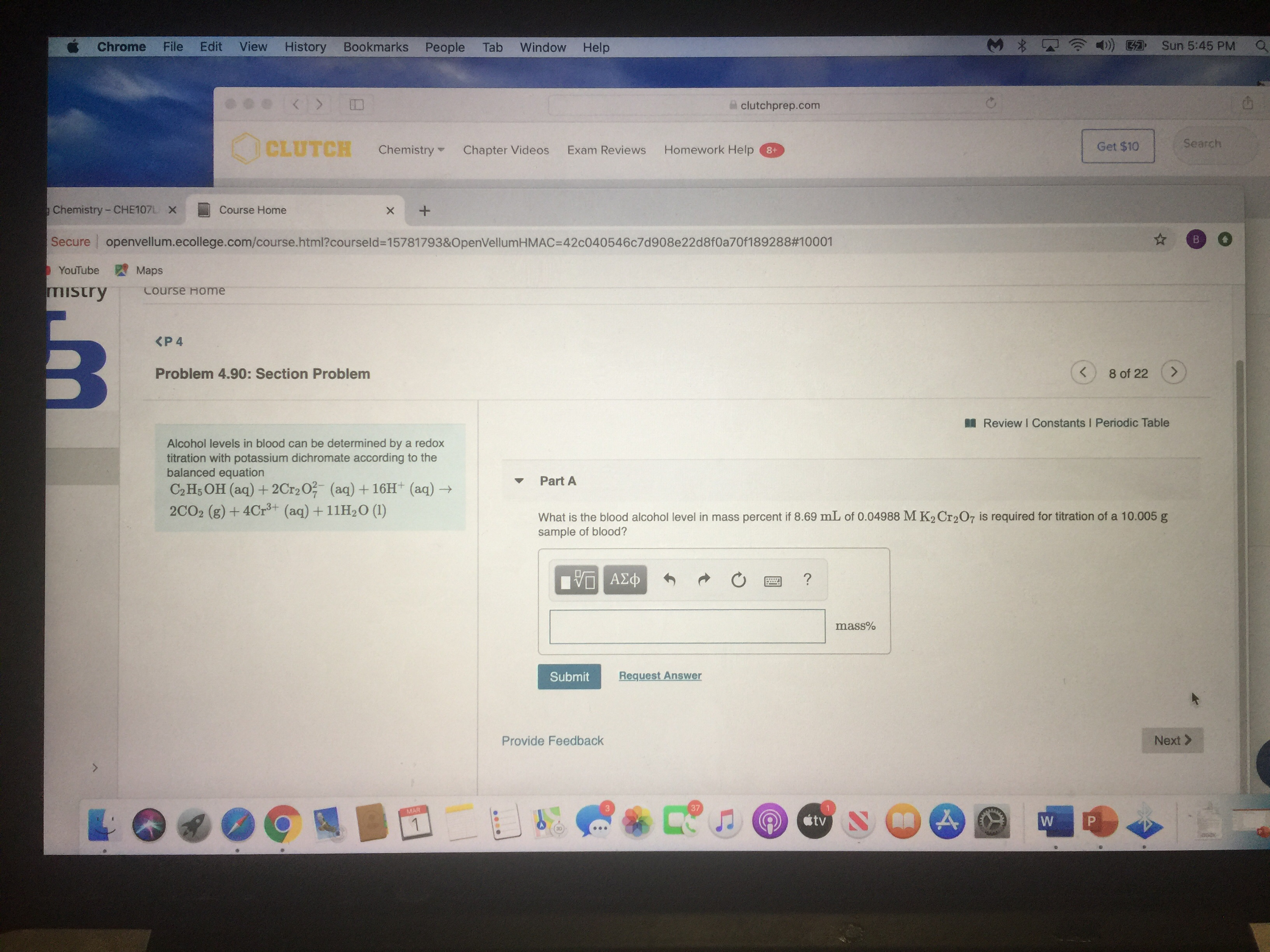# Alcohol levels in the blood can be determined by a redox titration with potassium dichromate according to the balanced equation: C2H5OH (aq) + 2Cr2O7^2- (aq) + 16 H^+ --> 2CO2 (g) + 4Cr^3+ (aq) + 11 H2O (l). What is the blood alcohol level in mass percent if 8.69 mL of 0.04988 M K2Cr2O7 is required for the titration of a 10.005 g sample of blood? Please solve this question for me. Thank you!